Learning Objective

In this lesson we will look more closely at density as a physical property, including what happens when substances with different densities are combined.

Learning Outcomes

By the end of this lesson you will be able to:

• Describe density.
• Express density as a mathematical relationship between mass and volume.
• Calculate the density of regular and irregular solids.
• Describe and explain the layering of substances with different densities.
• Explain how temperature and pressure can affect the density of a substance.

Density of Solids, Liquids and Gases

1 | Density

2 | Calculating Density

3 | Comparing Densities of Solids, Liquids and Gases

4 | Density Layering

5 | The Effect of Temperature on Density

6 | The Effect of Pressure on Density of Gases

7 | Summary

Click images to preview the worksheet for this lesson and the Year 8 Chemistry Workbook (PDF and print versions)

## Introduction

• In the lesson “Properties of Solids, Liquids and Gases”, we introduced density as a physical property, and looked at how it varies between the different states of matter.
• We saw that solids and liquids tend to have a high density, whereas gases tend to have a very low density.
• In the lesson “Particles in Solids, Liquids and Gases”, we learned that variation in density between solids, liquids and gases can be explained by the particle model.
• We saw that solids and liquids have high densities because their particles are packed closely together, whereas gases have low densities because their particles are very far apart.
• In this lesson we will look at how density is measured, and how substances with different densities interact.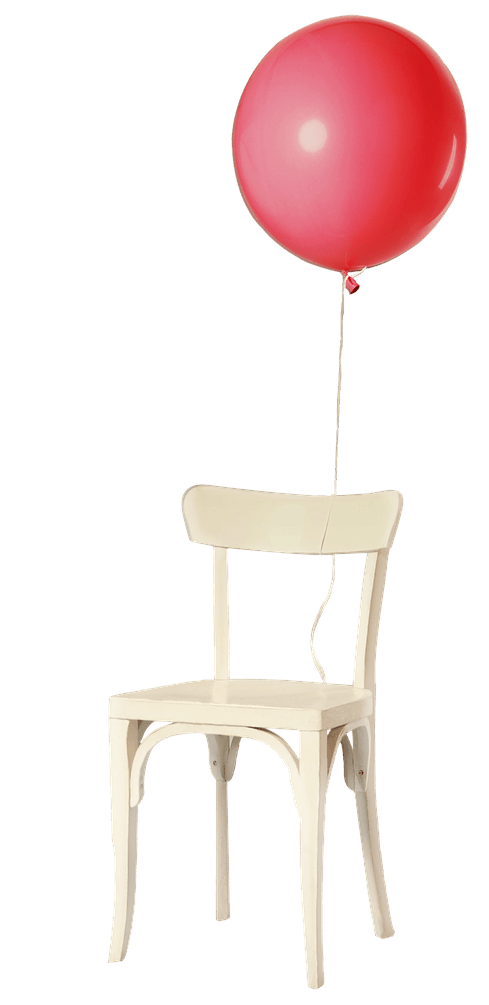(Image: Maria1968, Pixabay)

## Defining Density

• Previously, we described density as how heavy something is relative to its volume.
• In other words, density is a measure of how much matter is in a given amount of space.
• The amount of matter there is in a substance is referred to as its mass.
• The amount of space a substance occupies is referred to as its volume.
• Mass is measured in units such as grams (g) or kilograms (kg).
• Volume is measure in units such as cubic centimetres (cm3) or cubic metres (m3).
• Density can be expressed mathematically as:• Using symbols, this can be written as:• Density is measured in units such as grams per cubic centimetre (g/cm3) or kilograms per cubic metre (kg/m3).
• In a laboratory, g/cm3 is usually the most convenient unit for density.
• Therefore, when calculating density, mass must be in grams and volume must be in cubic centimetres.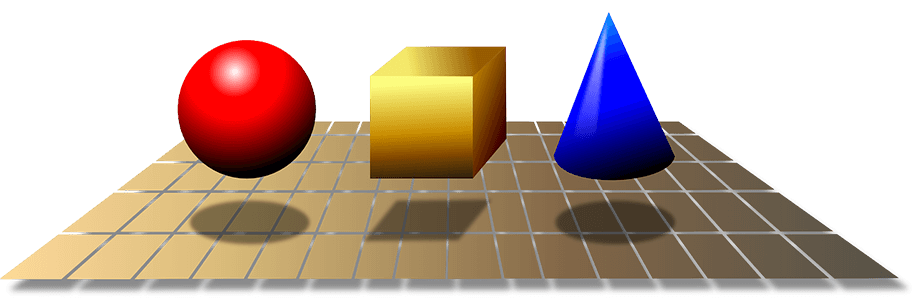Density refers to how much mass is in a given volume.

(Image: OpenClipart-Vectors, Pixabay)

## Calculating Density

• Given the above formula for density, to calculate the density of a particular substance, we need to first measure the mass and volume of a sample of that substance.

### Measuring Mass

• In a laboratory, small amounts of substances are weighed using an electronic balance.
• These give very accurate measurements, in grams, to 2 or 3 decimal places.
• It is important to make sure the balance is reading ‘zero’ before weighing something.
• If it isn’t reading zero, simple press the ‘tare’ button to reset the balance to zero.
• Often, substances need to be placed in a weighing tray or other vessel to be measured, as they can’t be placed directly on the balance. This includes substances such as powders and liquids.
• There are two methods for weighing substances in weighing trays, each involving a couple of additional steps.
• Method 1 (best for when you need to share the balance with others):
• Record the mass of the empty weighing tray.
• Place the substance in the weighing tray, then record the mass – this is the combined mass of the weighing tray and the substance.
• Calculate the mass of the substance by deducting the mass of the weighing tray (step 1) from the combined mass of the weighing tray and the substance (step 2).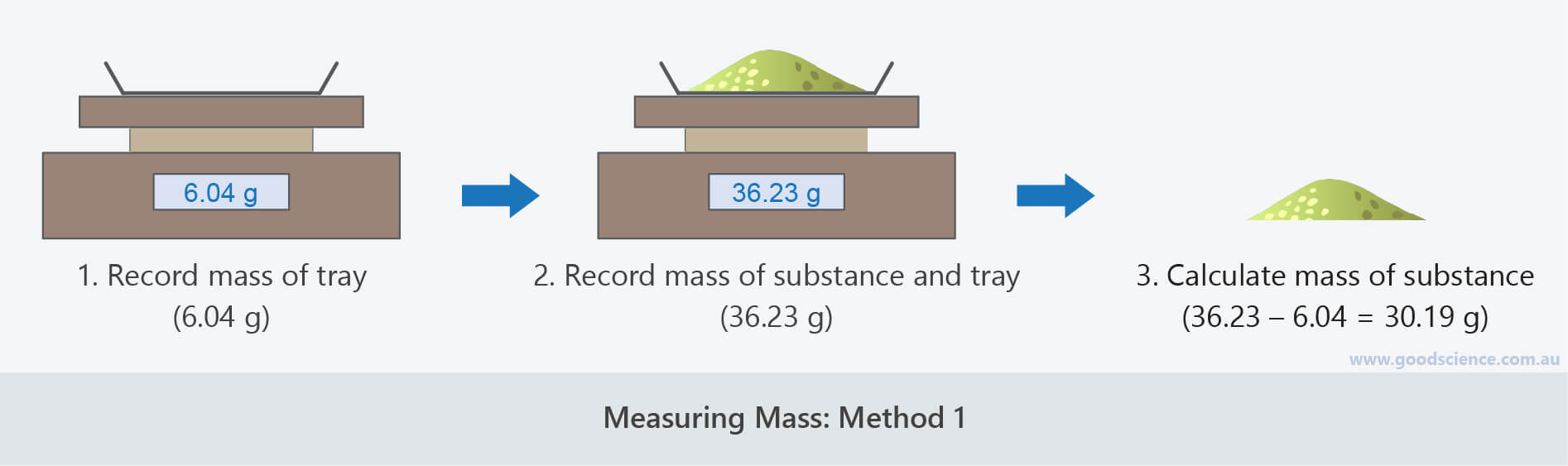This is the preferred method for measuring mass if you have to share a balance as you don’t need to zero the balance before collecting your sample.

• Method 2 (best for when you don’t need to share the balance with others):
• Place the empty weighing tray on the balance.
• Press the ‘tare’ button on the balance to ‘zero’ it.
• Place the substance on the weighing tray, then record the mass – this is the mass of the substance, as the mass of the weighing tray has already been deducted when the ‘tare’ button was pressed.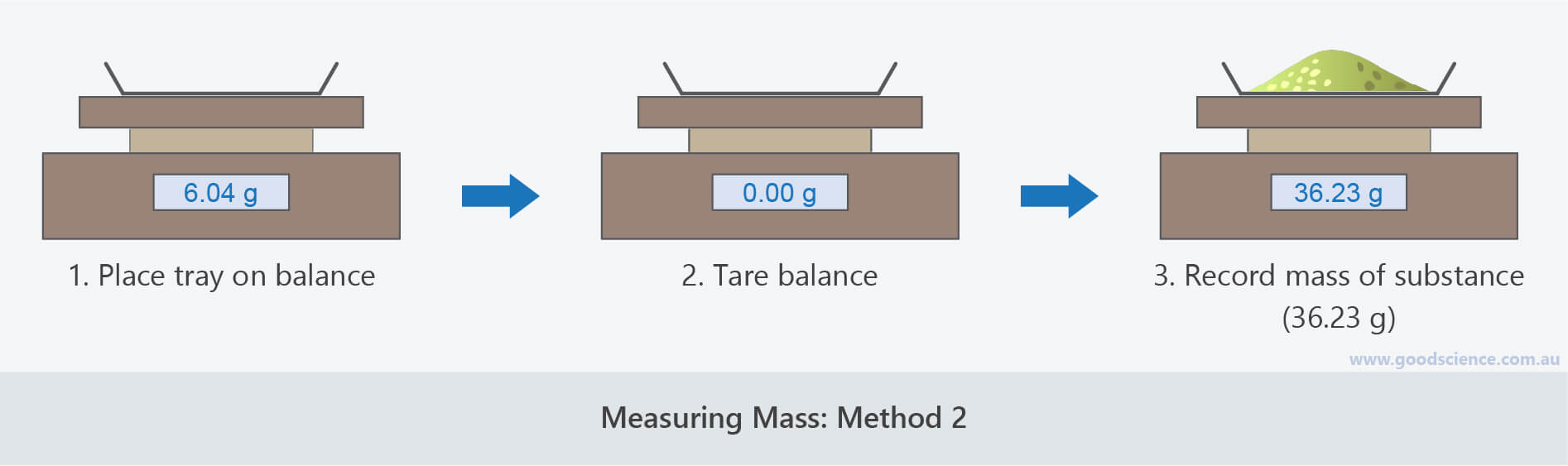This is the preferred method for measuring mass if you don’t have to share a balance as it requires no calculations.

### Measuring Volume

• The method for measuring the volume of a substance depends on the type of substance.

Liquids

• Liquids are the most straightforward substances for measuring volume.
• They are simply poured into a piece of laboratory glassware designed for measuring volume, such as a measuring cylinder.
• Laboratory glassware records volume in millilitres (mL), but this is easily converted to cubic centimetres by the following formula (which applies to water and most laboratory solutions):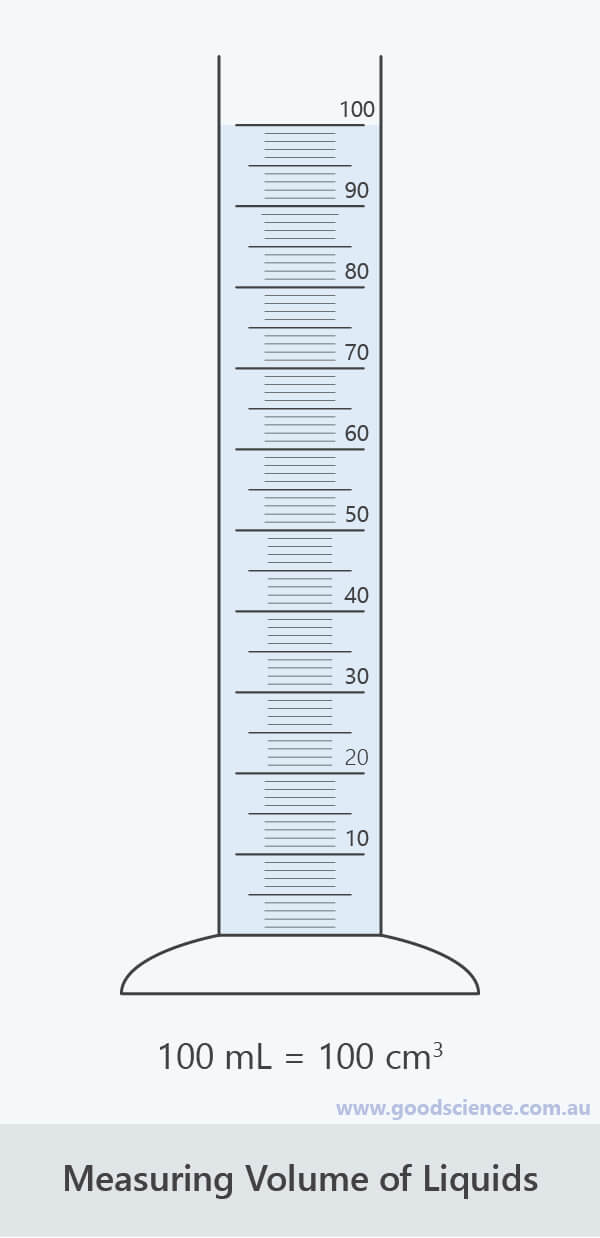The volume of a liquid in mL is equal to its volume in cm3.

Regular Solids

• The volume of regular solids, such as cubes, rectangular prisms, cylinders and spheres, can be calculated using mathematical formulas based on their dimensions – length, width and depth.
• For example, the volume of a rectangular prism can be calculated using the formula:• Using symbols, this can be written as:• The unit for volume will depend on the units used for measuring the dimensions.
• If the dimensions were measured in cm, the volume will be in cm3; if the dimensions were measured in m, the volume will be in m3, and so on.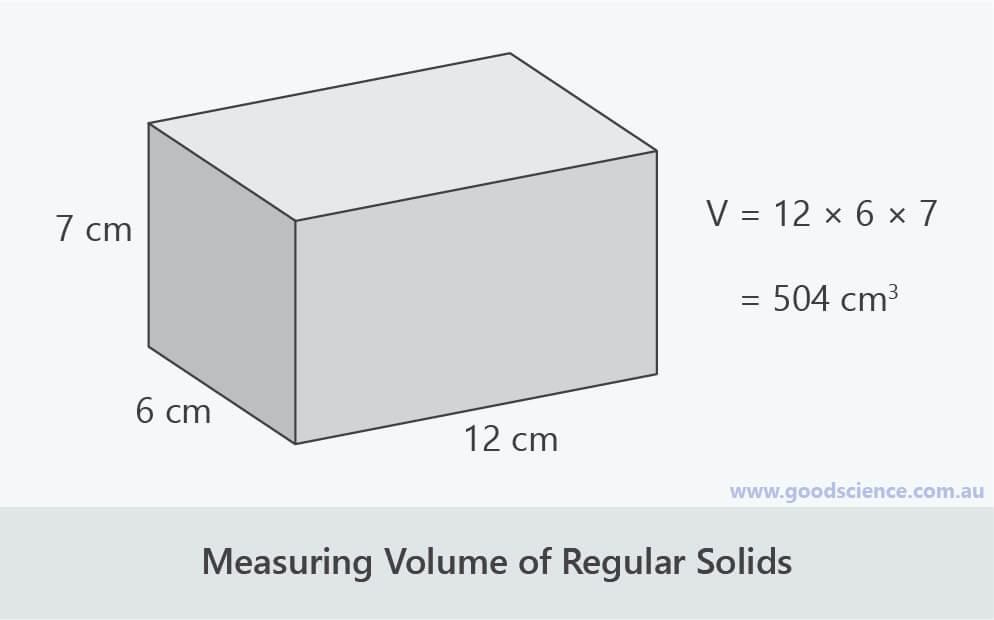The volume of regular solids can be calculated using mathematical formulas.

Irregular Solids

• Most solids do not have a regular shape, therefore, their volumes cannot be easily calculated using mathematical formulas.
• A simple alternative is to calculated their volumes based on the amount of liquid they displace.
• This involves the following method:
• Partially fill a measuring cylinder with water and record the volume.
• The cylinder needs to be wide enough that the solid being measured can fit in it.
• Also, the amount of space left in the measuring cylinder must be greater than the volume of the solid.
• If measuring hard solids, like rocks, use a plastic measuring cylinder rather than a glass one, to eliminate the risk of breakage.
• Carefully place the irregular solid into the measuring cylinder and record the new level of the water.
• This is the combined volume of the water and solid.
• Calculate the volume of the solid by deducting the volume of the water (step 1) from the combined volume of the water and solid (step 2).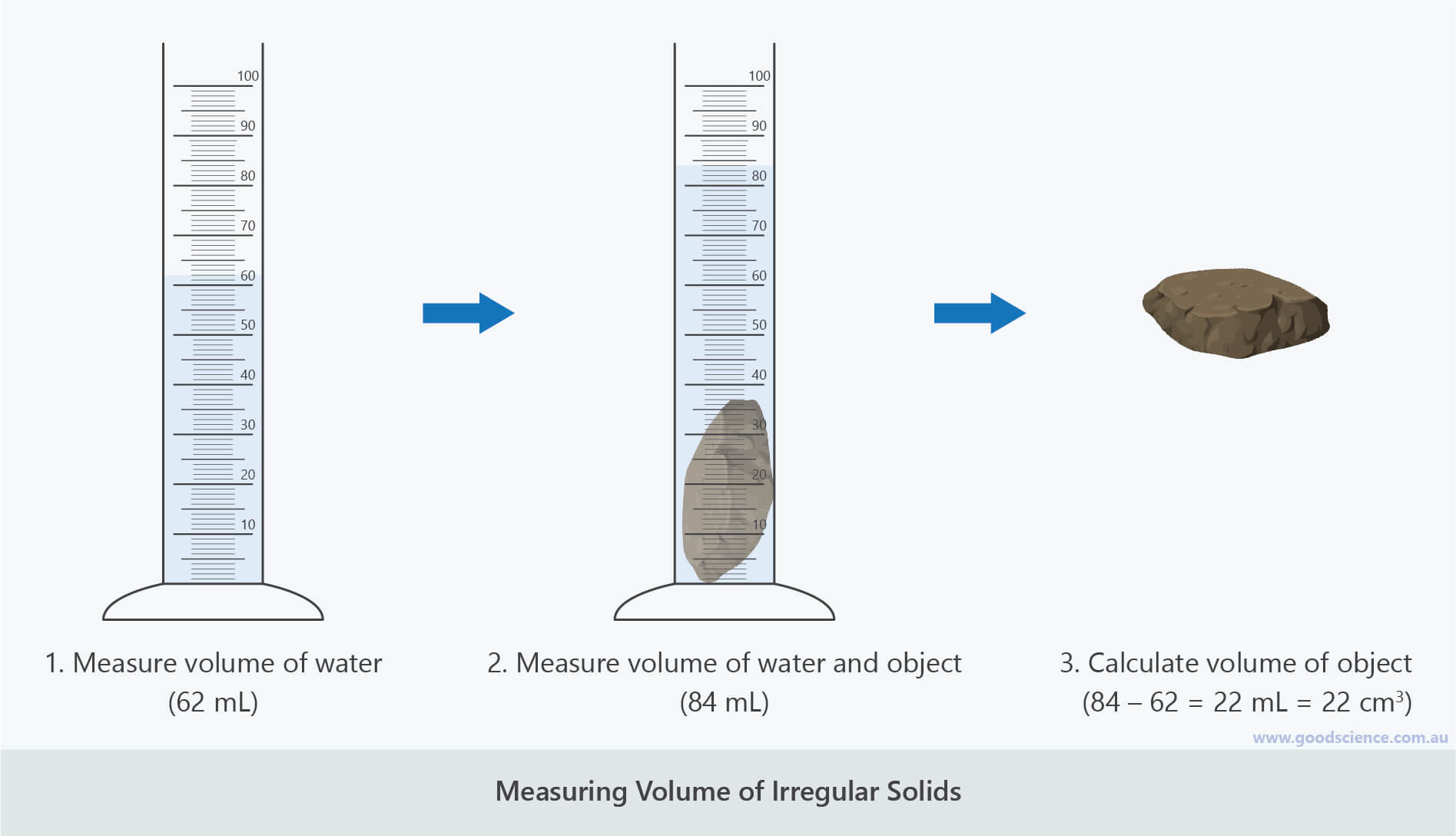The volume of irregular solids can be calculated by measuring their displacement of water.

## Comparing Densities of Solids, Liquids and Gases

• We can make some generalisations about the relative densities of solids, liquids and gases:
• Solids generally have high or very high densities.
• Liquids generally have high densities.
• Gases generally have very low densities.
• However, there is wide variation within each state of matter.
• For example, wood and lead have very different densities, even though they are both solids.
• Therefore, a piece of wood and a piece of lead the same size will have very different masses.
• The tables below show the densities of some different solids, liquids and gases.

•  Gas Density (g/cm3 at 25°C) Hydrogen 0.000081 Helium 0.00016 Nitrogen 0.0011 Oxygen 0.0013 Carbon dioxide 0.0018 Butane 0.0024 Sulfur hexafluoride 0.0062 Tungsten hexafluoride 0.010

Densities of different gases

•  Liquid Density (g/cm3 at 25°C) Petrol (gasoline) 0.73 Ethanol (alcohol) 0.79 Vegetable oil 0.91 Water 1.00 Seawater 1.03 Honey 1.36 Bromine 3.10 Mercury 13.6

Densities of different liquids

•  Solid Density (g/cm3 at 25°C) Wood (pine) 0.35 – 0.55 Sandstone 2.2 – 2.8 Glass 2.5 – 3.0 Aluminium 2.70 Diamond 3.52 Iron 7.87 Lead 11.3 Gold 19.3

Densities of different solids

## Density Layering

• Substances with different densities will form vertical layers, with an order that is related to the density of each substance.
• The least dense substance will sit at the top, while and the most dense substance will sit at the bottom.
• Example 1
• Water has a density of 1 g/cm3.
• Any substance with a density less than 1g/cm3, such as cooking oil or a cork, will float in water.
• Any substance with a density greater than 1g/cm3, such as honey or a stone, will sink in water.
• Example 2
• Air has a density of 0.0012 g/cm3.
• A balloon filled with a gas that is less dense than air, such as helium, will float away.
• A balloon filled with a gas that is more dense than air, such as sulfur hexafluoride, will fall to the ground.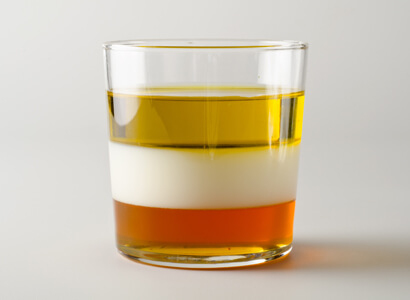A density tower (density column) consisting of cooking oil (top layer), milk (middle layer) and honey (bottom layer).

### Water and Ice

• Most substances contract slightly when they freeze, making them more dense.
• Therefore, when placed in liquid of the same substance, the frozen solid sinks.
• For example, if a piece of frozen cooking oil is placed in liquid cooking oil, it will sink.
• Water is unusual because it expands slightly when it freezes, making it less dense.
• Therefore, ice floats on water.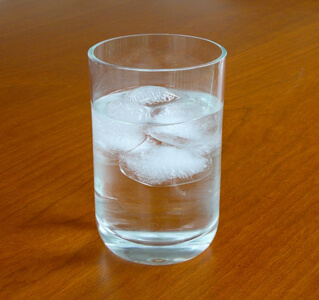Water is unusual because it is less dense as a solid than a liquid – therefore ice floats in water.

(Image: Victor Blacus, Wikimedia Commons)

## The Effect of Temperature on Density

• In the lesson “Expansion and Contraction in Solids, Liquids and Gases”, we learned that substances generally expand when heated and contract when cooled.
• We also learned that the volume of gases changes the most and the volume of solids changes the least.
• Therefore, since temperature affects the volume of a substance, it must also affect the density.
• An increase in temperature leads to an increase in volume and a decrease in density.
• An decrease in temperature leads to a decrease in volume and a increase in density.
• This principle is utilised in hot air balloons.
• By heating the air inside the balloon, it expands, becoming less dense than the surrounding air, causing the balloon to rise.Hot air balloons rise because warm air is less dense than cool air.

(Image: metaliza01, Pixabay)

## The Effect of Pressure on Density of Gases

• In the lesson “Properties of Solids, Liquids and Gases”, we learned that gases are easily compressed, whereas solids and liquids are not.
• This means that changes in pressure will affect the volume (and therefore density) of gases, but will not noticeably affect the volume (or density) of solids and liquids.
• For gases:
• An increase in pressure leads to a decrease in volume and an increase in density.
• A decrease in pressure leads to an increase in volume and an decrease in density.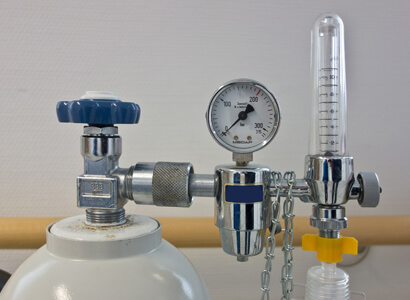Pressurising a gas increases its density.

(Image: blickpixel, Pixabay)

## Summary

• Density refers to how much matter is in a given amount of space.
• It can be expressed mathematically as:
•• where mass is the amount of matter in the substance and volume is the amount of space the substance occupies.
• Density is measured in units such as g/cm3 or kg/m3.
• The mass of a substance can be determined by weighing it on an electronic balance.
• The volume of a liquid can be determined by measuring it in a measuring cylinder.
• The volume of a regular solid can be determined using a mathematical formula.
• The volume of an irregular solid can be determined by measuring how much liquid it displaces in a measuring cylinder.
• Volumes measured in mL can be converted to cm3 using the formula:
•• Generally:
• Solids generally have high or very high densities.
• Liquids generally have high densities.
• Gases generally have very low densities.
• Mixtures containing substances with different densities will form vertical layers, where:
• The least dense substance will lie at the top.
• The most dense substance will lie at the bottom.
• Pure water has a density of 1 g/cm3.
• Therefore, any substance with a density less than 1g/cm3 will float in water and any substance with a density greater than 1g/cm3 will sink in water.
• Most frozen solids will sink in a liquid of the same substance as substances are usually more dense as solids than liquids.
• Ice is unusual because it is less dense than water and therefore floats in it.
• Heating substances causes them to expand and therefore become less dense.
• Cooling substances causes them to contract and therefore become more dense.
• Pressurising gases causes them to contract and therefore become more dense.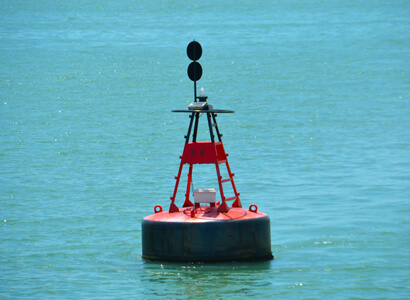(Image: terimakasih0, Pixabay)